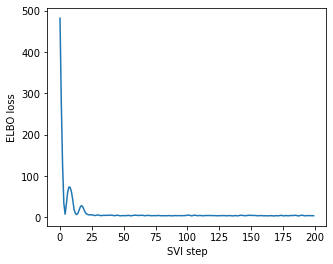# Infer_discrete with multiple sites and enumeration

I’m having trouble with a hierarchical mixture model, whose posterior inference is performed with autoguide and naive enumeration (I’m not confident about whether I’m enumerating properly). I’m showing a toy example that should be reproduceable, and raises the same error.

``````def toy_model(data=None, n1=3, n2=5):
p1 = pyro.param("p1", torch.randn(n1).exp(), constraint=constraints.simplex)
p2 = pyro.param("p2", torch.randn(n2).exp(), constraint=constraints.simplex)

with pyro.plate("level1", n1):
mu1 = pyro.sample("mu1", Normal(0, 1))

with pyro.plate("level2", n2):
z1 = pyro.sample("z1", Categorical(p1), infer={'enumerate': 'parallel'})
mu2 = pyro.sample("mu2", Normal(mu1[z1], 0.1))

with pyro.plate("data", N):
z2 = pyro.sample("z2", Categorical(p2), infer={'enumerate': 'parallel'}) #, infer={'enumerate': 'parallel'}
pyro.sample("obs", Normal(mu2[z2], 0.01), obs=data)

model = toy_model
``````

And the graph illustrates the hierarchical structure:

``````optim = ClippedAdam({'lr': 0.1, 'betas': [0.9, 0.999], 'lrd': 0.999})
elbo = pyro.infer.TraceEnum_ELBO(num_particles=4, max_plate_nesting=1)
def train(num_iterations, losses=[]):
for j in tqdm(range(num_iterations)):
loss = svi.step(data) / N
losses.append(loss)
return losses

def initialize(seed):
global global_guide, svi
pyro.set_rng_seed(seed)
pyro.clear_param_store()

global_guide = pyro.infer.autoguide.AutoDelta(
poutine.block(model, hide=['z1', 'z2']),
)
svi = SVI(model, global_guide, optim, loss=elbo)
return svi.loss(model, global_guide, data) / N

loss, seed = min((initialize(seed), seed) for seed in tqdm(range(100)))
initialize(seed)
print('seed = {}, initial_loss = {}'.format(seed, loss))

losses = []
losses = train(200, losses=losses)
``````

ELBO loss converges:Here comes the problem: when I’m directly following the patterns here to perform MAP inference for discrete sites

``````guide_trace = poutine.trace(global_guide).get_trace(data)  # record the globals
trained_model = poutine.replay(model, trace=guide_trace)  # replay the globals

def classifier(data, temperature=0): #random assignment if temperature=1
inferred_model = infer_discrete(trained_model, temperature=temperature,
first_available_dim=-2)  # avoid conflict with data plate

trace = poutine.trace(inferred_model).get_trace(data)
return trace.nodes["z2"]["value"]
classifier(data)
``````

I got an error

``````ValueError: Error while packing tensors at site 'mu2':
Invalid tensor shape.
Allowed dims: -1
Actual shape: (3, 5)
Try adding shape assertions for your model's sample values and distribution parameters.
Trace Shapes:
Param Sites:
p1     3
p2     5
Sample Sites:
mu1 dist   3 |
value   3 |
log_prob   3 |
z1 dist   5 |
value 3 1 |
log_prob 3 5 |
mu2 dist 3 5 |
value   5 |
log_prob 3 5 |
Trace Shapes:
Param Sites:
Sample Sites:
``````

The `infer_discrete` and `classifier` worked well for programs with only one discrete site, and after checking the shape, I thought the error should be related with enumeration. How should I deal with this issue?

Perhaps any tips on such kind of indexing? Seems that I often get into trouble during enumerationThe error is about packing sites:

``````e:\00NSFC-categorization\00_experiment-Categorization\B02_REFRESH.ipynb Cell 21 in classifier(data, temperature)
5 def classifier(data, temperature=0): #random assignment if temperature=1
6     inferred_model = infer_discrete(trained_model, temperature=temperature,
7                                     first_available_dim=-2)  # avoid conflict with data plate
----> 8     trace = poutine.trace(inferred_model).get_trace(data)
9     return trace.nodes["z"]["value"]

File c:\Users\19046\.conda\envs\pytorch\lib\site-packages\pyro\poutine\trace_messenger.py:198, in TraceHandler.get_trace(self, *args, **kwargs)
190 def get_trace(self, *args, **kwargs):
191     """
192     :returns: data structure
193     :rtype: pyro.poutine.Trace
(...)
196     Calls this poutine and returns its trace instead of the function's return value.
197     """
--> 198     self(*args, **kwargs)
199     return self.msngr.get_trace()

File c:\Users\19046\.conda\envs\pytorch\lib\site-packages\pyro\poutine\trace_messenger.py:180, in TraceHandler.__call__(self, *args, **kwargs)
178         exc = exc_type("{}\n{}".format(exc_value, shapes))
179         exc = exc.with_traceback(traceback)
--> 180         raise exc from e
182         "_RETURN", name="_RETURN", type="return", value=ret
183     )
184 return ret

File c:\Users\19046\.conda\envs\pytorch\lib\site-packages\pyro\poutine\trace_messenger.py:174, in TraceHandler.__call__(self, *args, **kwargs)
171     "_INPUT", name="_INPUT", type="args", args=args, kwargs=kwargs
172 )
173 try:
--> 174     ret = self.fn(*args, **kwargs)
175 except (ValueError, RuntimeError) as e:
176     exc_type, exc_value, traceback = sys.exc_info()

File c:\Users\19046\.conda\envs\pytorch\lib\site-packages\pyro\infer\discrete.py:51, in _sample_posterior(model, first_available_dim, temperature, strict_enumeration_warning, *args, **kwargs)
49 enum_trace = prune_subsample_sites(enum_trace)
50 enum_trace.compute_log_prob()
---> 51 enum_trace.pack_tensors()
53 return _sample_posterior_from_trace(
54     model, enum_trace, temperature, strict_enumeration_warning, *args, **kwargs
55 )

File c:\Users\19046\.conda\envs\pytorch\lib\site-packages\pyro\poutine\trace_struct.py:429, in Trace.pack_tensors(self, plate_to_symbol)
427 _, exc_value, traceback = sys.exc_info()
428 shapes = self.format_shapes(last_site=site["name"])
--> 429 raise ValueError(
430     "Error while packing tensors at site '{}':\n  {}\n{}".format(
431         site["name"], exc_value, shapes
432     )
433 ).with_traceback(traceback) from e
``````

The most confusing part is here: It seems OK to have z1 site enumerated, but the downstream shape covaries with it, and I think this is the cause. Sadly I have no idea how to fix this. Could any one help me please? I’ve been struck here for some weeksI’ve hit the same bug couple of years agoThere is a solution that I tried that worked for me. Maybe it will work for you as well1 Like

Oh, I see!I’ll try it out. Thanks a lot!